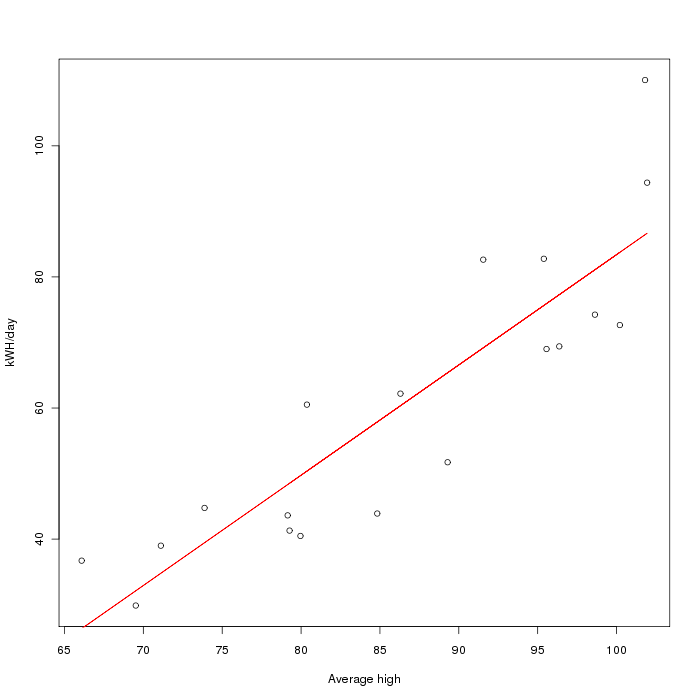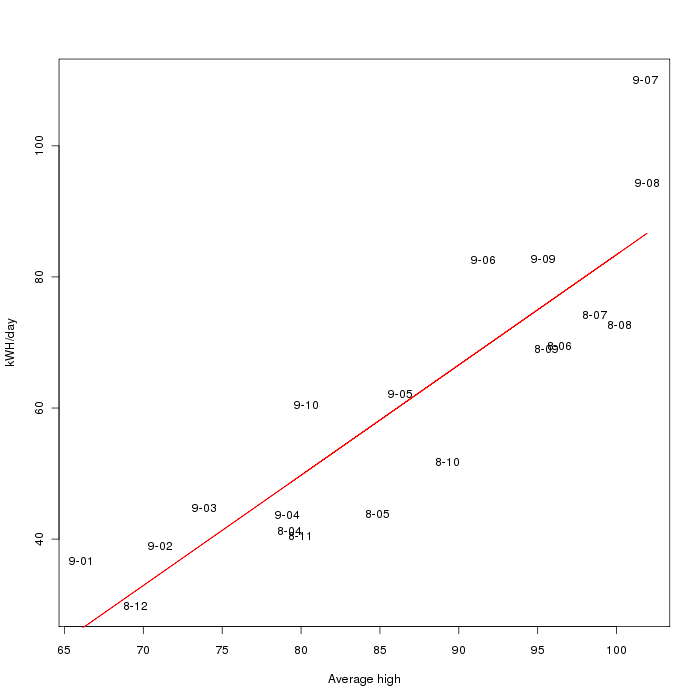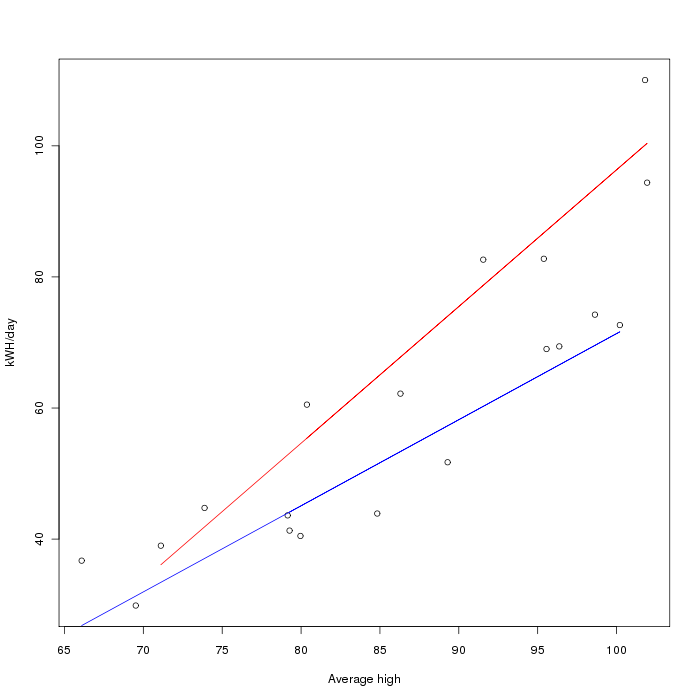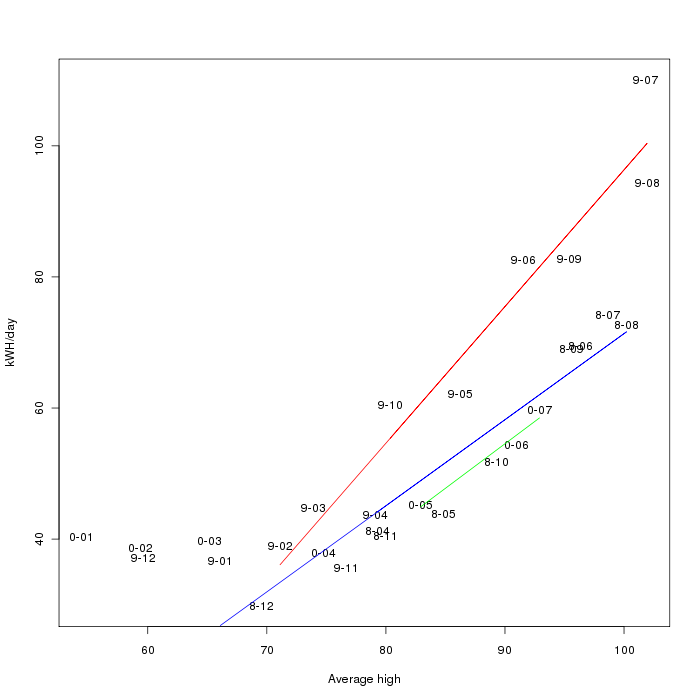Determining home energy usage from temperature

New: Here's an updated visualization, made with Tableau Public:

I was curious about how our home energy usage varied with respect to temperature, so I made some graphs with R. The first graph is a graph of the average high temperature in Austin versus our kWH/day energy usage (see where I got the data from) with a fitted linear regression line. The slope is 1.68, indicating that for every degree hotter it gets, we spend 1.68 kWH extra per day.However, the line is not a great fit for the data (R-squared is 0.7778), so I decided to look at a plot with the dates (in "year-month") on it:Aha! It looks like there's something else going on - almost all the 2009 entries are above the line, and all the 2008 entries are below the line. If we split these up into two regressions, we get:The blue line (for 2008) has slope 1.31 with R-squared 0.8979, and the red line (for 2009) has slope 2.086 with R-squared 0.9344.

Updated graph with new months:About this project: The temperature data was taken from a few places: firstly this infochimps data set, augmented with the preliminary reports from the National Climatic Data Center and the monthly reports from Camp Mabry.

For the energy data, I didn't have all of our old Austin Energy bills, but I did know how much they were for so I was going to estimate based on that. This turned out to be somewhat inaccurate, but I was able to sign up for an online account and get all the old data, which was extremely helpful since it had the kWH/day averages. (since billing cycles seem to range between 28 and 35(!) days)

After processing that, I loaded it into R, and did the following analyzes/plots:

• Plotting the raw data:
plot(temps[], kwh[], xlab="Average high", ylab="kWH/day")
• Running and plotting the first linear regression:
l <- lm(kwh[] ~ temps[])
plot(temps[], kwh[], xlab="Average high", ylab="kWH/day")
points(temps[], l\$fit, type='l', col='red')
• Plotting the year-month data:
plot(temps[], kwh[], xlab="Average high", ylab="kWH/day", type='n')
text(temps[], kwh[], labels=substr(temps[], 4, 7), xlab="Average high", ylab="kWH/day")

The output of the regressions was:

• Regression with all:
> summary(l)

Call:
lm(formula = kwh[] ~ temps[])

Residuals:
Min      1Q  Median      3Q     Max
-13.972  -7.556  -2.249   7.421  23.587

Coefficients:
Estimate Std. Error t value Pr(>|t|)
(Intercept) -84.8509    19.0008  -4.466  0.00034 ***
temps[]    1.6825     0.2181   7.713 5.98e-07 ***
---
Signif. codes:  0 '***' 0.001 '**' 0.01 '*' 0.05 '.' 0.1 ' ' 1

Residual standard error: 10.66 on 17 degrees of freedom
Multiple R-squared: 0.7778,     Adjusted R-squared: 0.7647
F-statistic: 59.49 on 1 and 17 DF,  p-value: 5.98e-07
• Regression of 2008 data:
> summary(lPre)

Call:
lm(formula = kwh[][1:10] ~ temps[][1:10])

Residuals:
Min      1Q  Median      3Q     Max
-7.5246 -4.1163 -0.2126  3.3127  9.9011

Coefficients:
Estimate Std. Error t value Pr(>|t|)
(Intercept)      -59.9954    13.5826  -4.417  0.00224 **
temps[][1:10]   1.3135     0.1566   8.388  3.1e-05 ***
---
Signif. codes:  0 '***' 0.001 '**' 0.01 '*' 0.05 '.' 0.1 ' ' 1

Residual standard error: 5.694 on 8 degrees of freedom
Multiple R-squared: 0.8979,     Adjusted R-squared: 0.8851
F-statistic: 70.36 on 1 and 8 DF,  p-value: 3.101e-05
• Regression for 2009:
> summary(lPost)

Call:
lm(formula = kwh[][11:19] ~ temps[][11:19])

Residuals:
Min     1Q Median     3Q    Max
-9.233 -5.572  2.896  3.918  9.919

Coefficients:
Estimate Std. Error t value Pr(>|t|)
(Intercept)       -112.2145    18.2743  -6.141 0.000472 ***
temps[][11:19]    2.0855     0.2088   9.987 2.16e-05 ***
---
Signif. codes:  0 '***' 0.001 '**' 0.01 '*' 0.05 '.' 0.1 ' ' 1

Residual standard error: 6.812 on 7 degrees of freedom
Multiple R-squared: 0.9344,     Adjusted R-squared: 0.9251
F-statistic: 99.74 on 1 and 7 DF,  p-value: 2.158e-05